Anúncio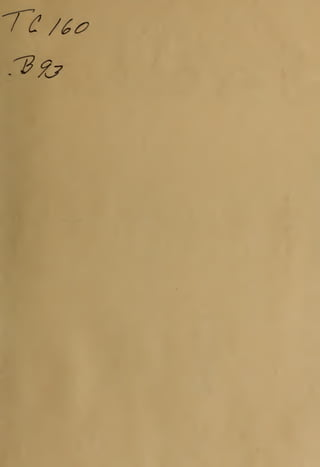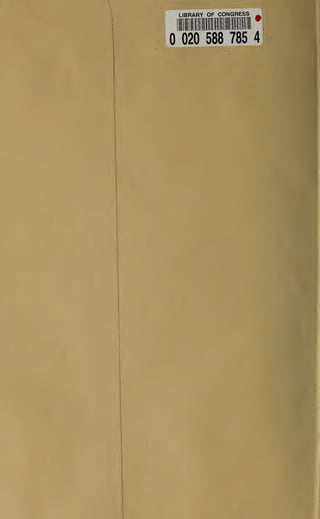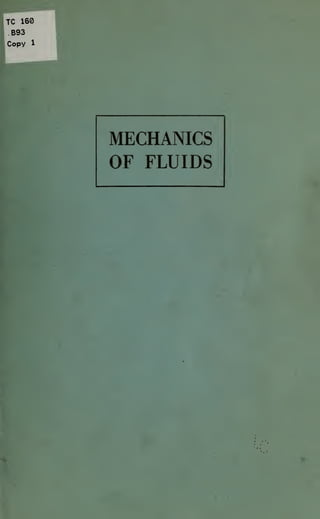Anúncio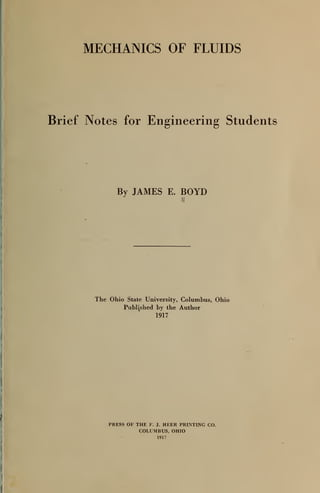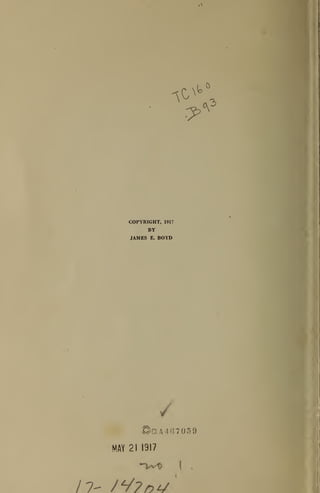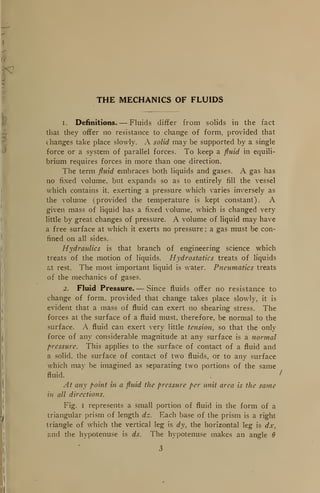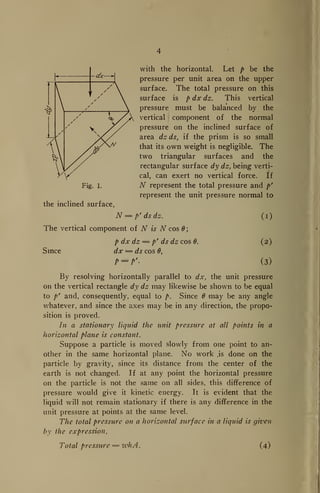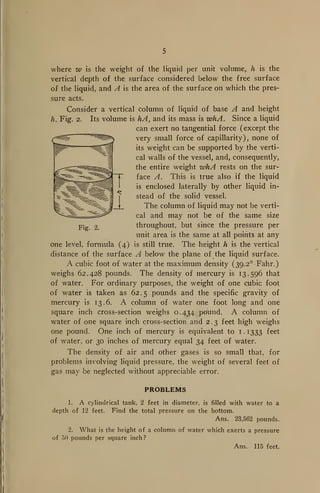Anúncio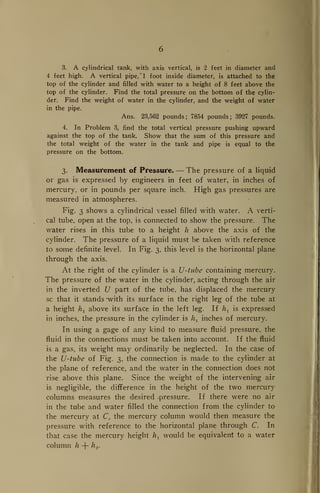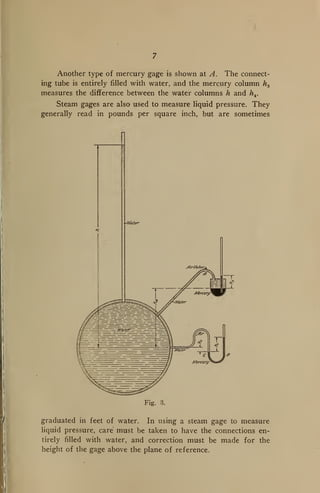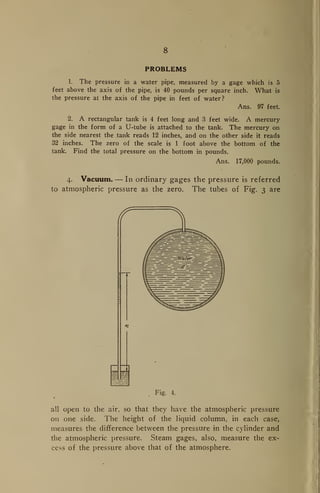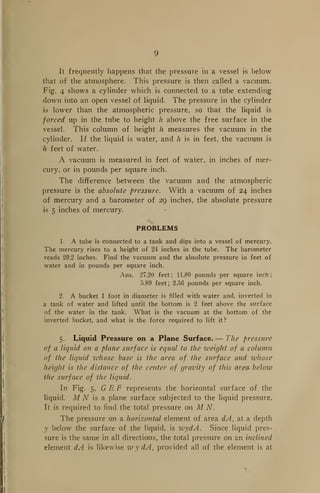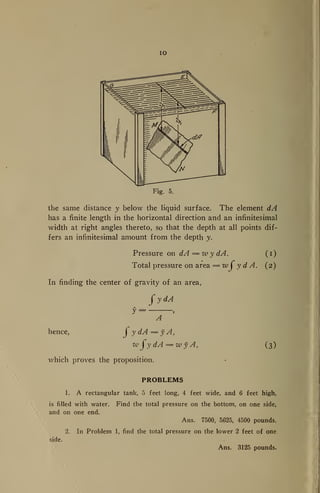Anúncio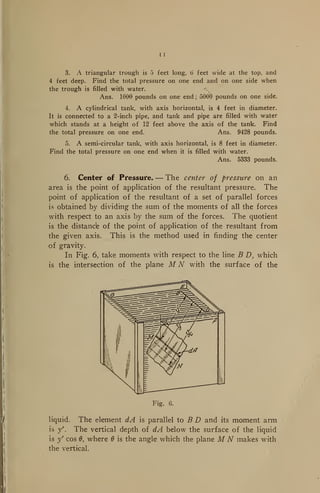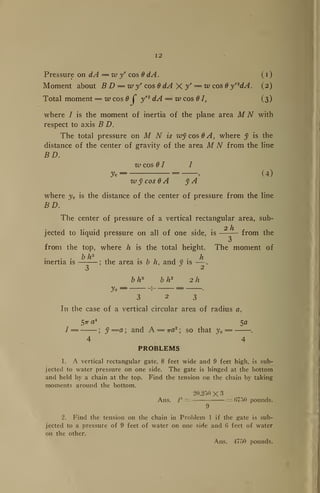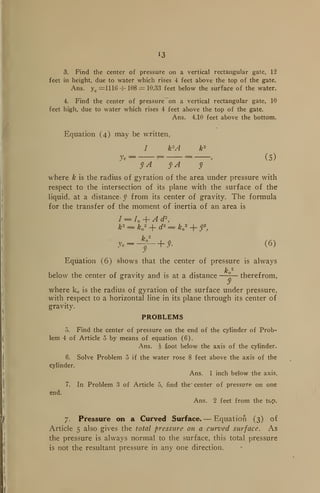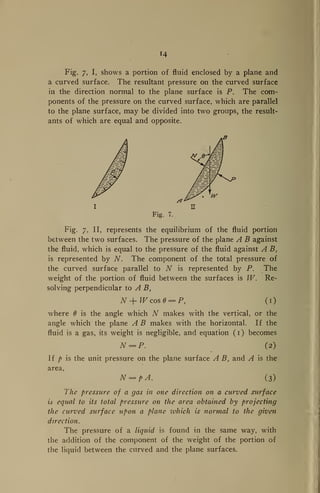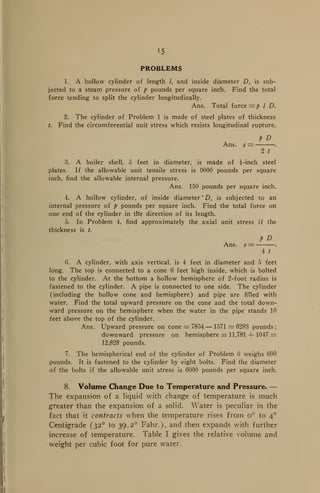Anúncio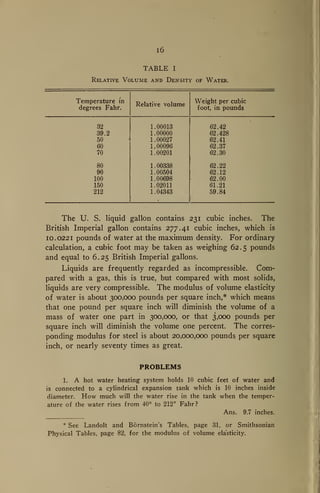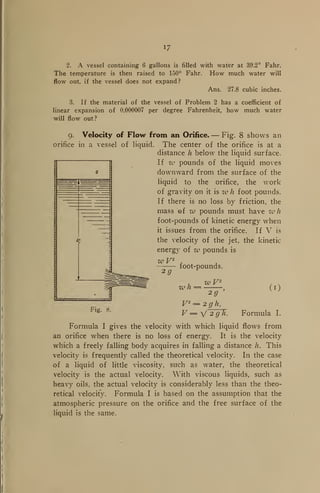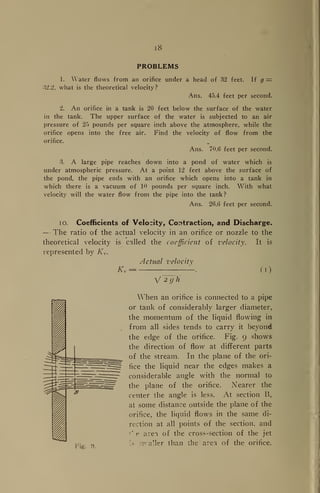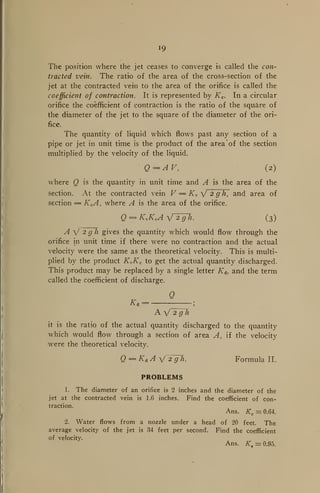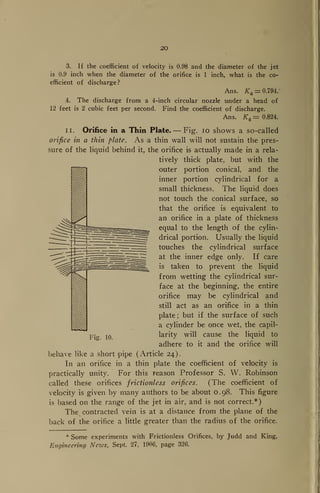Anúncio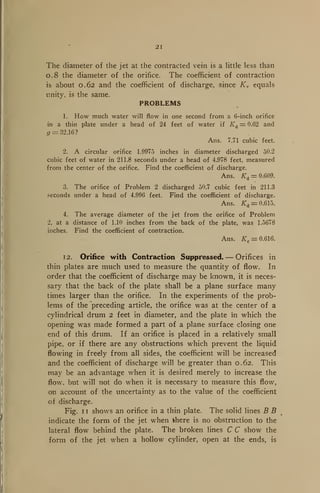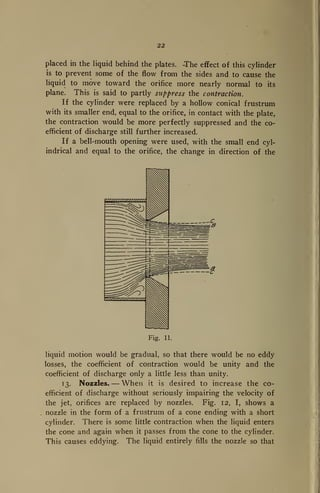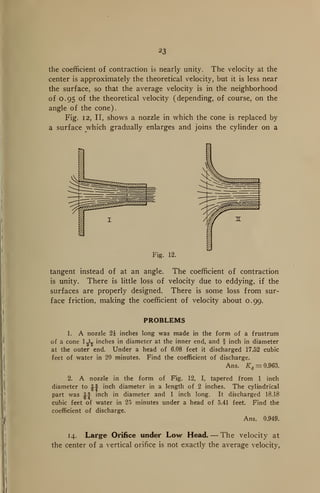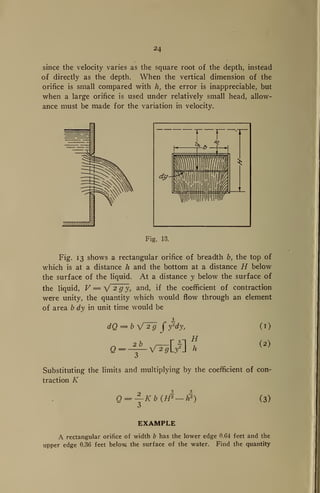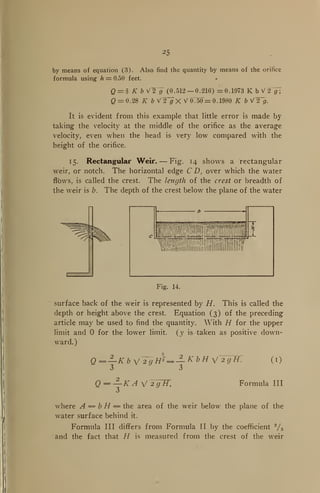Anúncio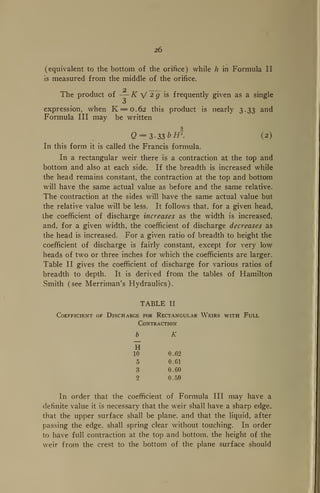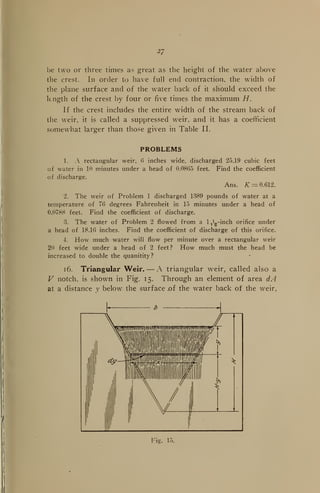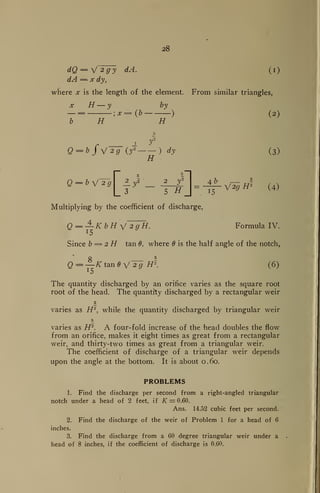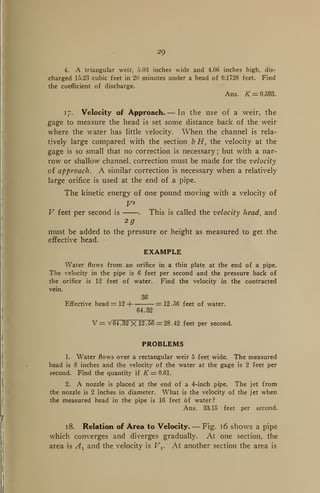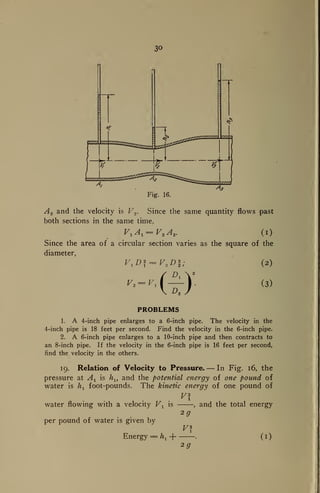Anúncio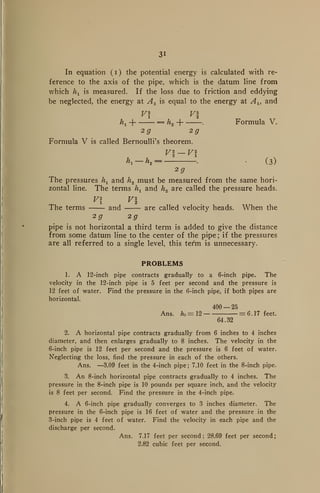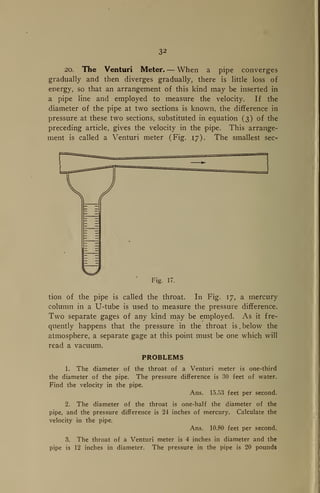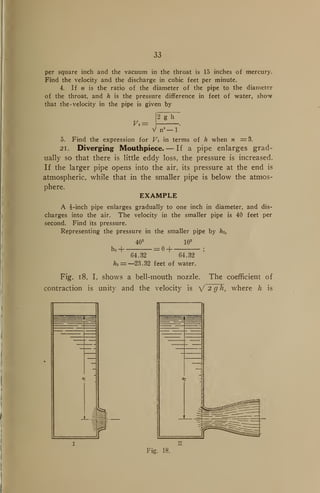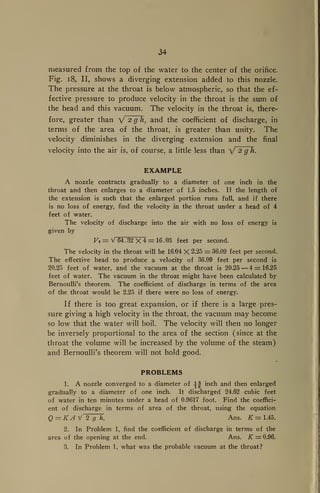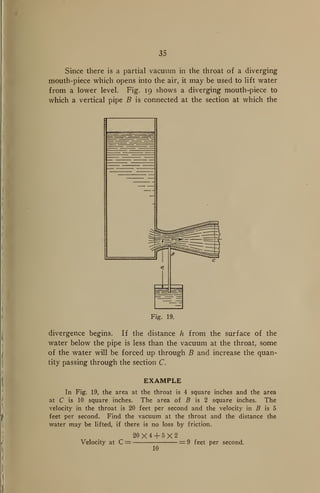Anúncio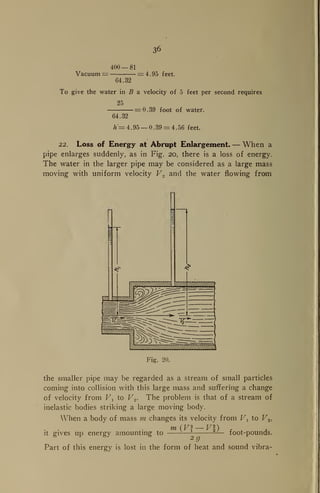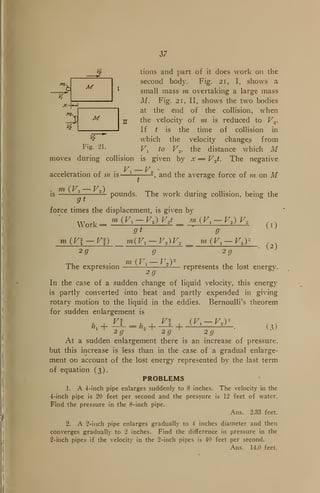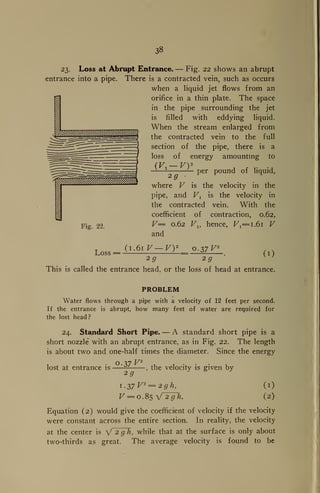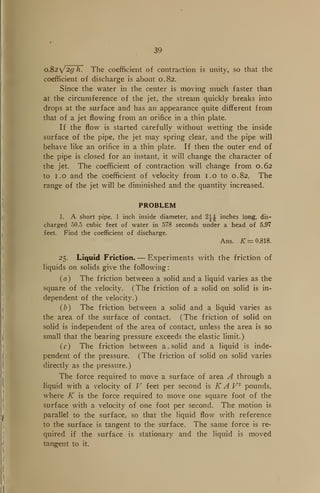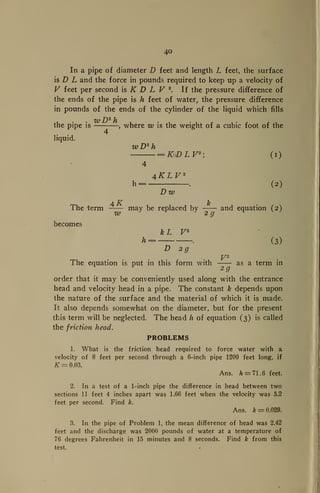Anúncio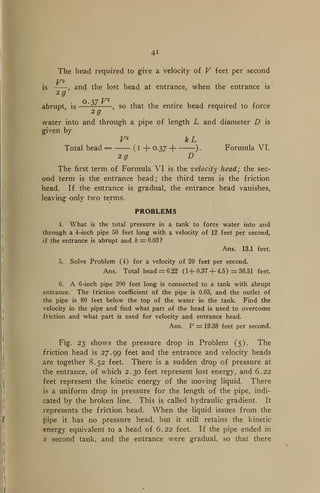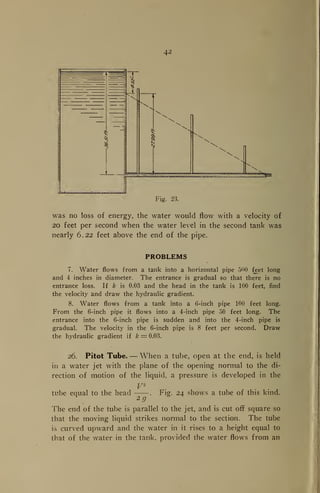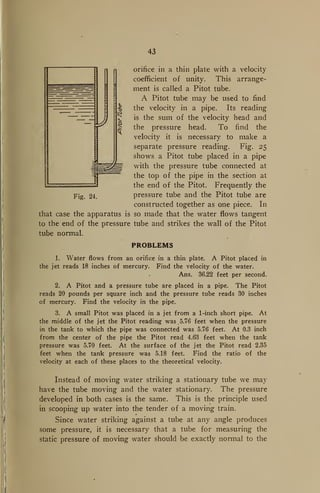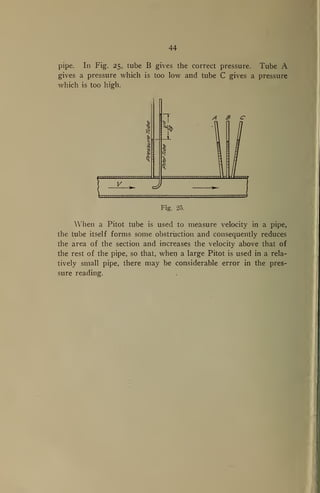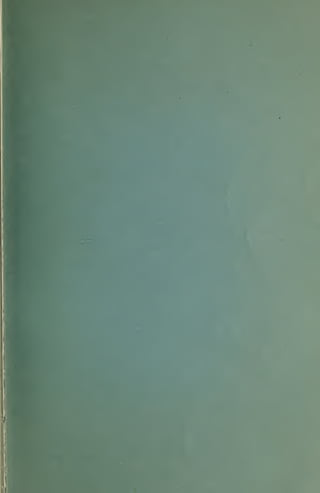Próximos SlideShares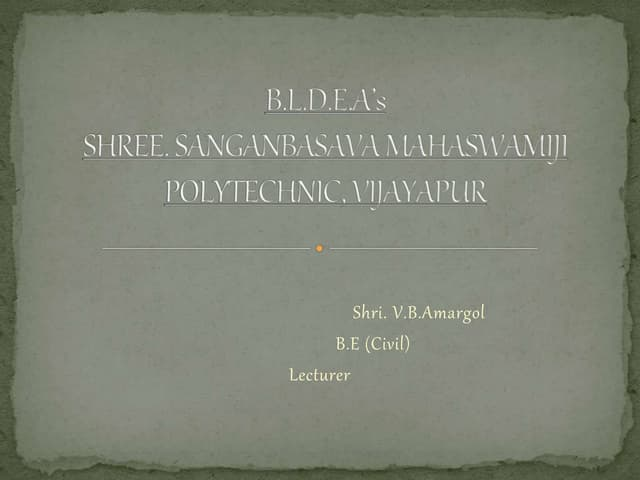PROPERTIES OF FLUIDS & ITS PRESSURE MEASURMENTS
Carregando em ... 3
1 de 50
Anúncio

### Mechanics of fluids

1. LIBRARY OF. CONGRESS ^ 020 588 785 4
2. TC 160 B93 Copy 1 MECHANICS OF FLUIDS
3. MECHANICS OF FLUIDS Brief Notes for Engineering Students By JAMES E. BOYD The Ohio State University, Columbus, Ohio Published by the Author 1917 PRESS OF THE F. J. HEER PRINTING CO. COLUMRUS, OHIO 1917
4. ^k° ^ COPYRIGHT, 1917 BY JAMES E. BOYD / ©C!,A4()7059 MAY 21 1917 11- J^?f>4^
5. THE MECHANICS OF FLUIDS i. Definitions. — Fluids differ from solids in the fact that they offer no resistance to change of form, provided that changes take place slowly. A solid may be supported by a single force or a system of parallel forces. To keep a fluid in equili- brium requires forces in more than one direction. The term fluid embraces both liquids and gases. A gas has no fixed volume, but expands so as to entirely fill the vessel which contains it, exerting a pressure which varies inversely as the volume (provided the temperature is kept constant). A given mass of liquid has a fixed volume, which is changed very little by great changes of pressure. A volume of liquid may have a free surface at which it exerts no pressure ; a gas must be con- fined on all sides. Hydraulics is that branch of engineering science which treats of the motion of liquids. Hydrostatics treats of liquids at rest. The most important liquid is water. Pneumatics treats of the mechanics of gases. 2. Fluid Pressure. — Since fluids offer no resistance to change of form, provided that change takes place slowly, it is evident that a mass of fluid can exert no shearing stress. The forces at the surface of a fluid must, therefore, be normal to the surface. A fluid can exert very little tension, so that the only force of any considerable magnitude at any surface is a normal pressure. This applies to the surface of contact of a fluid and a solid, the surface of contact of two fluids, or to any surface which may be imagined as separating two portions of the same fluid. At any point in a fluid the pressure per unit area is the same in all directions. Fig. i represents a small portion of fluid in the form of a triangular prism of length dz. Each base of the prism is a right triangle of which the vertical leg is dy, the horizontal leg is dx, and the hypotenuse is ds. The hypotenuse makes an angle 6
6. Fig. 1. the inclined surface, with the horizontal. Let p be the pressure per unit area on the upper surface. The total pressure on this surface is p dx dz. This vertical pressure must be balanced by the vertical component of the normal pressure on the inclined surface of area dz ds, if the prism is so small that its own weight is negligible. The two triangular surfaces and the rectangular surface dy dz, being verti- cal, can exert no vertical force. If N represent the total pressure and p' represent the unit pressure normal to N = p' dsdz. The vertical component of N is N cos 6 ; p dx dz = p' ds dz cos 0. Since dx = ds cos 0, p = p (i) (2) (3) By resolving horizontally parallel to dx, the unit pressure on the vertical rectangle dy dz may likewise be shown to be equal to p f and, consequently, equal to p. Since may be any angle whatever, and since the axes may be in any direction, the propo- sition is proved. In a stationary liquid the unit pressure at all points in a horizontal plane is constant. Suppose a particle is moved slowly from one point to an- other in the same horizontal plane. No work ,is done on the particle by gravity, since its distance from the center of the earth is not changed. If at any point the horizontal pressure on the particle is not the same on all sides, this difference of pressure would give it kinetic energy. It is evident that the liquid will not remain stationary if there is any difference in the unit pressure at points at the same level. The total pressure on a horizontal surface in a liquid is given by the expression, Total pressure = zvhA, (4)
7. where w is the weight of the liquid per unit volume, h is the vertical depth of the surface considered below the free surface of the liquid, and A is the area of the surface on which the pres- sure acts. Consider a vertical column of liquid of base A and height h. Fig. 2. Its volume is hA, and its mass is whA. Since a liquid can exert no tangential force (except the very small force of capillarity), none of its weight can be supported by the verti- cal walls of the vessel, and, consequently, the entire weight whA rests on the sur- face A. This is true also if the liquid is enclosed laterally by other liquid in- stead of the solid vessel. The column of liquid may not be verti- cal and may not be of the same size throughout, but since the pressure per unit area is the same at all points at any one level, formula (4) is still true. The height h is the vertical distance of the surface A below the plane of the liquid surface. A cubic foot O'f water at the maximum density (39.2 Fahr.) weighs 62.428 pounds. The density of mercury is 13.596 that of water. For ordinary purposes, the weight of one cubic foot of water is taken as 62.5 pounds and the specific gravity of mercury is 13.6. A column of water one foot long and one square inch cross-section weighs 0.434 pound. A column of water of one square inch cross-section and 2.3 feet high weighs one pound. One inch of mercury is equivalent to 1.1333 feet of water, or 30 inches of mercury equal 34 feet of water. The density of air and other gases is so small that, for problems involving liquid pressure, the weight of several feet of gas may be neglected without appreciable error. Fig. 2. PROBLEMS 1. A cylindrical tank, 2 feet in diameter, is filled with water to a depth of 12 feet. Find the total pressure on the bottom. Ans. 23,562 pounds. 2. What is the height of a column of water which exerts a pressure of 50 pounds per square inch? Ans. 115 feet.
8. 3. A cylindrical tank, with axis vertical, is 2 feet in diameter and 4 feet high. A vertical pipe, 1 foot inside diameter, is attached to the top of the cylinder and filled with water to a height of 8 feet above the top of the cylinder. Find the total pressure on the bottom of the cylin- der. Find the weight of water in the cylinder, and the weight of water in the pipe. Ans. 23,562 pounds; 7854 pounds; 3927 pounds. 4. In Problem 3, find the total vertical pressure pushing upward against the top of the tank. Show that the sum of this pressure and the total weight of the water in the tank and pipe is equal to the pressure on the bottom. 3. Measurement of Pressure. — The pressure of a liquid or gas is expressed by engineers in feet of water, in inches of mercury, or in pounds per square inch. High gas pressures are measured in atmospheres. Fig. 3 shows a cylindrical vessel filled with water. A verti- cal tube, open at the top, is connected to show the pressure. The water rises in this tube to a height h above the axis of the cylinder. The pressure of a liquid must be taken with reference to some definite level. In Fig. 3, this level is the horizontal plane through the axis. At the right of the cylinder is a U-tube containing mercury. The pressure of the water in the cylinder, acting through the air in the inverted U part of the tube, has displaced the mercury sc that it stands -with its surface in the right leg of the tube at a height h 1 above its surface in the left leg. If h 1 is expressed in inches, the pressure in the cylinder is h inches of mercury. In using a gage of any kind to measure fluid pressure, the fluid in the connections must be taken into account. If the fluid is a gas, its weight may ordinarily be neglected. In the case of the U-tube of Fig. 3, the connection is made to the cylinder at the plane of reference, and the water in the connection does not rise above this plane. Since the weight of the intervening air is negligible, the difference in the height of the two mercury columns measures the desired .pressure. If there were no air in the tube and water filled the connection from the cylinder to the mercury at C, the mercury column would then measure the pressure with reference to the horizontal plane through C. In that case the mercury height h x would be equivalent to a water column h -- h 2 .
9. Another type of mercury gage is shown at A. The connect- ing tube is entirely filled with water, and the mercury column hz measures the difference between the water columns h and h±. Steam gages are also used to measure liquid pressure. They generally read in pounds per square inch, but are sometimes -V&ter graduated in feet of water. In using a steam gage to measure liquid pressure, care must be taken to have the connections en- tirely filled with water, and correction must be made for the height of the gage above the plane of reference.
10. PROBLEMS 1. The pressure in a water pipe, measured by a gage which is 5 feet above the axis of the pipe, is 40 pounds per square inch. What is the pressure at the axis of the pipe in feet of water? Ans. 97 feet. 2. A rectangular tank is 4 feet long and 3 feet wide. A mercury gage in the form of a U-tube is attached to the tank. The mercury on the side nearest the tank reads 12 inches, and on the other side it reads 32 inches. The zero of the scale is 1 foot above the bottom of the tank. Find the total pressure on the bottom in pounds. Ans. 17,000 pounds. 4. Vacuum. — In ordinary gages the pressure is referred to atmospheric pressure as the zero. The tubes of Fig. 3 are all open to the air, so that they have the atmospheric pressure on one side. The height of the liquid column, in each case, measures the difference between the pressure in the cylinder and the atmospheric pressure. Steam gages, also, measure the ex- cess of the pressure above that of the atmosphere.
11. It frequently happens that the pressure in a vessel is below that of the atmosphere. This pressure is then called a vacuum. Fig. 4 shows a cylinder which is connected to a tube extending down into an open vessel of liquid. The pressure in the cylinder is lower than the atmospheric pressure, so that the liquid is forced up in the tube to height h above the free surface in the vessel. This column of height h measures the vacuum in the cylinder. If the liquid is water, and h is in feet, the vacuum is h feet of water. A vacuum is measured in feet of water, in inches of mer- cury, or in pounds per square inch. The difference between the vacuum and the atmospheric pressure is the absolute pressure. With a vacuum of 24 inches of mercury and a barometer of 29 inches, the absolute pressure is 5 inches of mercury. PROBLEMS 1. A tube is connected to a tank and dips into a vessel of mercury. The mercury rises to a height of 24 inches in the tube. The barometer reads 29.2 inches. Find the vacuum and the absolute pressure in feet of water and in pounds per square inch. Ans. 27.20 feet; 11.80 pounds per square inch; 5.89 feet ; 2.56 pounds per square inch. 2. A bucket 1 foot in diameter is filled with water and, inverted in a tank of water and lifted until the bottom is 2 feet above the surface of the water in the tank. What is the vacuum at the bottom of the inverted bucket, and what is the force required to lift it? 5. Liquid Pressure on a Plane Surface. — The pressure of a liquid on a plane surface is equal to the weight of a column of the liquid whose base is the area of the surface and whose height is the distance of the center of gravity of this area below the surface of the liquid. In Fig. 5, G E F represents the horizontal surface of the liquid. M N is a plane surface subjected to the liquid pressure. It is required to find the total pressure on M N. The pressure on a horizontal element of area dA, at a depth y below the surface of the liquid, is zvydA. Since liquid pres- sure is the same in all directions, the -total pressure on an inclined element dA is likewise w y dA, provided all of the element is at
12. 10 Fig. 5. the same distance y below the liquid surface. The element dA has a finite length in the horizontal direction and an infinitesimal width at right angles thereto, so that the depth at all points dif- fers an infinitesimal amount from the depth y. Pressure on dA = iv y dA. ( I Total pressure on area = wfydA. (2) In finding the center of gravity of an area, CydA hence, C y dA = y A, w jy dA = w y A, which proves the proposition. (3) PROBLEMS 1. A rectangular tank, 5 feet long, 4 feet wide, and 6 feet high, is filled with water. Find the total pressure on the bottom, on one side, and on one end. Ans. 7500, 5625, 450O pounds. 2. In Problem 1, find the total pressure on the lower 2 feet of one side. Ans. 3125 pounds.
13. 1 I 3. A triangular trough is 5 feet long, 6 feet wide at the top, and 4 feet deep. Find the total pressure on one end and on one side when the trough is filled with water. Ans. 1000 pounds on one end ; 5000 pounds on one side. 4. A cylindrical tank, with axis horizontal, is 4 feet in diameter. It is connected to a 2-inch pipe, and tank and pipe are filled with water which stands at a height of 12 feet above the axis of the tank. Find the total pressure on one end. Ans. 9428 pounds. 5. A semi-circular tank, with axis horizontal, is 8 feet in diameter. Find the total pressure on one end when it is filled with water. Ans. 5333 pounds. 6. Center of Pressure. — The center of pressure on an area is the point of application of the resultant pressure. The point of application of the resultant of a set of parallel forces is obtained by dividing the sum of the moments of all the forces with respect to an axis by the sum of the forces. The quotient is the distance of the point of application of the resultant from the given axis. This is the method used in finding the center of gravity. In Fig. 6, take moments with respect to the line B D, which is the intersection of the plane M N with the surface of the Fig. 6. liquid. The element dA is parallel to B D and its moment arm is y'. The vertical depth of dA below the surface of the liquid is y' cos 6, where 6 is the angle which the plane M N makes with the vertical.
14. 12 Pressure on dA — w y' cos OdA. (i) Moment about B D = wy' cos OdA X / = w cos 6y'2 dA. (2) Total moment = w cos 6 C y'2 dA = w cos 1, (3) where / is the moment of inertia of the plane area M N with respect to axis B D. The total pressure on M N is wy cos A, where y is the distance of the center of gravity of the area M N from the line BD. w cos 01 I yc = = , (4) w y cos A y A where yc is the distance of the center of pressure from the line BD. The center of pressure of a vertical rectangular area, sub- 2 h jected to liquid pressure on all of one side, is from the o from the top, where h is the total height. The moment of b hs h inertia is ; the area is b h, and y is - —3 2 b h* b h2 2 h yc = ; = . 323In the case of a vertical circular area of radius a, 5tt a4 5a / = ; y —a ; and A = tto 2 ; so that yc = PROBLEMS 1. A vertical rectangular gate, 8 feet wide and 9 feet high, is sub- jected to water pressure on one side. The gate is hinged at the bottom and held by a chain at the top. Find the tension on the chain by taking moments around the bottom. 20,250X3 Ans. P = " ()750 pounds. 9 2. Find the tension on the chain in Problem 1 if the gate is sub- jected to a pressure of 9 feet of water on one side and 6 feet of water on the other. Ans. 4750 pounds.
15. 13 3. Find the center of pressure on a vertical rectangular gate, 12 feet in height, due to water which rises 4 feet above the top of the gate. Ans. yc =1116 -v- 108 = 10.33 feet below the surface of the water. 4. Find the center of pressure on a vertical rectangular gate, 10 feet high, due to water which rises 4 feet above the top of the gate. Ans. 4.10 feet above the bottom. Equation (4) may be written, / k 2 A k 2 3'c = = = , (5) y A y A y where k is the radius of gyration of the area under pressure with respect to the intersection of its plane with the surface of the liquid, at a distance y from its center of gravity. The formula for the transfer of the moment of inertia of an area is / = I + A d k 2 = k 2 + d2 = k 2 + f, Vc = -|^ + ^ (6) Equation (6) shows that the center of pressure is always k 2 below the center of gravity and is at a distance —° — therefrom, where k is the radius of gyration of the surface under pressure, with respect to a horizontal line in its plane through its center of gravity. PROBLEMS 5. Find the center of pressure on the end of the cylinder of Prob- lem 4 of Article 5 by means of equation (6). Ans. i foot below the axis of the cylinder. 6. Solve Problem 5 if the water rose 8 feet above the axis of the cylinder. Ans. 1 inch below the axis. 7. In Problem 3 of Article 5, find the' center of pressure on one end. Ans. 2 feet from the top. 7. Pressure on a Curved Surface. — Equation (3) of Article 5 also gives the total pressure on a curved surface. As the pressure is always normal to the surface, this total pressure is not the resultant pressure in any one direction.
16. 14 Fig. 7, I, shows a portion of fluid enclosed by a plane and a curved surface. The resultant pressure on the curved surface in the direction normal to the plane surface is P. The com- ponents of the pressure on the curved surface, which are parallel to the plane surface, may be divided into two groups, the result- ants of which are equal and opposite. Fig. 7. Fig- 7, II, represents the equilibrium of the fluid portion between the two surfaces. The pressure of the plane A B against the fluid, which is equal to the pressure of the fluid against A B, is represented by N. The component of the total pressure of the curved surface parallel to N is represented by P. The weight of the portion of fluid between the surfaces is W. Re- solving perpendicular to A B, N+WcosO = P, (i) where 6 is the angle which N makes with the vertical, or the angle which the plane A B makes with the horizontal. If the fluid is a gas, its weight is negligible, and equation (i) becomes N = P. (2) If p is the unit pressure on the plane surface A B, and A is the area, N — pA. (3) The pressure of a gas in one direction on a curved surface is equal to its total pressure on the area obtained by projecting the curved surface upon a plane which is normal to the given direction. The pressure of a liquid is found in the same way, with the addition of the component of the weight of the portion of the liquid between the curved and the plane surfaces.
17. i5 PROBLEMS 1. A hollow cylinder of length /, and inside diameter D, is sub- jected to a steam pressure of p pounds per square inch. Find the total force tending to split the cylinder longitudinally. Ans. Total force = p I D. 2. The cylinder of Problem 1 is made of steel plates of thickness t. Find the circumferential unit stress which resists longitudinal rupture. P D Ans. s = . 2 t 3. A boiler shell, 5 feet in diameter, is made of i-inch steel plates. If the allowable unit tensile stress is 9000 pounds per square inch, find the allowable internal pressure. Ans. 150 pounds per square inch. 4. A hollow cylinder, of inside diameter K D, is subjected to an internal pressure of p pounds per square inch. Find the total force on one end of the cylinder in trfe direction of its length. 5. In Problem 4, find approximately the axial unit stress if the thickness is t. Ans. j : 6. A cylinder, with axis vertical, is 4 feet in diameter and 5 feet long. The top is connected to a cone 6 feet high inside, which is bolted to the cylinder. At the bottom a hollow hemisphere of 2-foot radius is fastened to the cylinder. A pipe is connected to one side. The cylinder (including the hollow cone and hemisphere) and pipe are filled with water. Find the total upward pressure on the cone and the total down- ward pressure on the hemisphere when the water in the pipe stands 10 feet above the top of the cylinder. Ans. Upward pressure on cone = 7854 — 1571 = 6283 pounds ; downward pressure on hemisphere = 11,781 -4- 1047 = 12,828 pounds. 7. The hemispherical end of the cylinder of Problem 6 weighs 600 pounds. It is fastened to the cylinder by eight bolts. Find the diameter of the bolts if the allowable unit stress is 6000 pounds per square inch. 8. Volume Change Due to Temperature and Pressure, — The expansion of a liquid with change of temperature is much greater than the expansion of a solid. Water is peculiar in the fact that it contracts when the temperature rises from o° to 4 Centigrade (32 to 39. 2° Fahr.), and then expands with further increase of temperature. Table I gives the relative volume and weight per cubic foot for pure water.
18. i6 TABLE I Relative Volume and Density of Water. Temperature in degrees Fahr. Relative volume Weight per cubic foot, in pounds 32 1.00013 62.42 39.2 1.00000 62.428 50 I 1.00027 62.41 60 1,00096 62.37 70 1.00201 62.30 80 1.00338 62.22 90 1.00504 ! 62.12 100 1.00698 62.00 150 1.02011 61.21 212 1.04343 59.84 The U. S. liquid gallon contains 231 cubic inches. The British Imperial gallon contains 277.41 cubic inches, which is 10.0221 pounds of water at the maximum density. For ordinary calculation, a cubic foot may be taken as weighing 62.5 pounds and equal to 6.25 British Imperial gallons. Liquids are frequently regarded as incompressible. Com- pared with a gas, this is true, but compared with most solids, liquids are very compressible. The modulus of volume elasticity of water is about 300,000 pounds per square inch,* which means that one pound per square inch will diminish the volume of a mass of water one part in 300,000, or that 3,000 pounds per square inch will diminish the volume one percent. The corres- ponding modulus for steel is about 20,000,000 pounds, per square inch, or nearly seventy times as great. PROBLEMS 1. A hot water heating system holds 10 cubic feet of water and is connected to a cylindrical expansion tank which is 10 inches inside diameter. How much will the water rise in the tank when the temper- ature of the water rises from 40° to 212° Fahr? Ans. 9.7 inches. * See Landolt and Bornstein's Tables, page 31, or Smithsonian Physical Tables, page 82, for the modulus of volume elasticity.
19. 17 2. A vessel containing 6 gallons is filled with water at 39.2° Fahr. The temperature is then raised to 150° Fahr. How much water will flow out, if the vessel does not expand? Ans. 27.8 cubic inches. 3. If the material of the vessel of Problem 2 has a coefficient of linear expansion of 0.000007 per degree Fahrenheit, how much water will flow out? 9. Velocity of Flow from an Orifice. — Fig. 8 shows an orifice in a vessel of liquid. The center of the orifice is at a distance h below the liquid surface. If w pounds of the liquid moves downward from the surface of the liquid to the orifice, the work of gravity on it is w h foot pounds. If there is no loss by friction, the mass of w pounds must have w h foot-pounds of kinetic energy when it issues from the orifice. If V is the velocity of the jet, the kinetic energy of w pounds is wV2 *g Fig. 8. foot-pounds. wV2 ft = 29 V2 = 2gh, 7c' V = V 2 g h. Co Formula I. Formula I gives the velocity with which liquid flows from an orifice when there is no loss of energy. It is the velocity which a freely falling body acquires in falling a distance h. This velocity is frequently called the theoretical velocity. In the case of a liquid of little viscosity, such as water, the theoretical velocity is the actual velocity. With viscous liquids, such as heavy oils, the actual velocity is considerably less than the theo- retical velocity. Formula I is based on the assumption that the atmospheric pressure on the orifice and the free surface of the liquid is the same.
20. i8 PROBLEMS 1. Water flows from an orifice under a head of 32 feet. Ii g = 32.2, what is the theoretical velocity? Ans. 45.4 feet per second. 2. An orifice in a tank is 20 feet below the surface of the water in the tank. The upper surface of the water is subjected to an air pressure of 25 pounds per square inch above the atmosphere, while the orifice opens into the free air. Find the velocity of flow from the orifice. Ans. 70.6 feet per second. 3. A large pipe reaches down into a pond of water which is under atmospheric pressure. At a point 12 feet above the surface of the pond, the pipe ends with an orifice which opens into a tank in which there is a vacuum of 10 pounds per square inch. With what velocity will the water flow from the pipe into the tank? Ans. 26.6 feet per second. io. Coefficients of Velocity, Contraction, and Discharge. —- The ratio of the actual velocity in an orifice or nozzle to the theoretical velocity is called the coefficient of velocity. It is represented by Ky . Actual velocity K, = — — ( i V 2 g h Fig. !». When an orifice is connected to a pipe or tank of considerably larger diameter, the momentum of the liquid flowing in from all sides tends to carry it beyond the edge of the orifice. Fig. 9 shows the direction of flow at different parts of the stream. In the plane of the ori- fice the liquid near the edges makes a considerable angle with the normal to the plane of the orifice. Nearer the center the angle is less. At section B, at some distance outside the plane of the orifice, the liquid flows in the same di- rection at all points of the section, and t 1 e area of the cross-section of the jet is smaller than the area of the orifice.
21. 19 The position where the jet ceases to converge is called the con- tracted vein. The ratio of the area of the cross-section of the jet at the contracted vein to the area of the orifice is called the coefficient of contraction. It is represented by Kc . In a circular orifice the coefficient of contraction is the ratio of the square of the diameter of the jet to the square of the diameter of the ori- fice. The quantity of liquid which flows past any section of a pipe or jet in unit time is the product of the area of the section multiplied by the velocity of the liquid. Q=AV, (2) where is the quantity in unit time and A is the area of the section. At the contracted vein V = Kv / 2 g h, and area of section = KC A, where A is the area of the orifice. Q = Kx Kc Ay 2gh. (3) A / 2 g h gives the quantity which would flow through the orifice in unit time if there were no contraction and the actual velocity were the same as the theoretical velocity. This is multi- plied by the product KVKC to get the actual quantity discharged. This product may be replaced by a single letter K&, and the term called the coefficient of discharge. Q Kd = ; A V 2 gh it is the ratio of the actual quantity discharged to the quantity which would flow through a section of area A, if the velocity were the theoretical velocity. Q = Kd A ^f~2gh. Formula II. PROBLEMS 1. The diameter of an orifice is 2 inches and the diameter of the jet at the contracted vein is 1.6 inches. Find the coefficient of con- traction. Ans. Kc = 0.64. 2. Water flows from a nozzle under a head of 20 feet. The average velocity of the jet is 34 feet per second. Find the coefficient of velocity. Ans. Kr = 0.95.
22. 20 3. If the coefficient of velocity is 0.98 and the diameter of the jet is 0.9 inch when the diameter of the orifice is 1 inch, what is the co- efficient of discharge? Ans. Kd = 0.794. 4. The discharge from a 4-inch circular nozzle under a head of 12 feet is 2 cubic feet per second. Find the coefficient of discharge. Ans. Kd = 0.824. ii. Orifice in a Thin Plate. — Fig. io shows a so-called orifice in a thin plate. As a thin wall will not sustain the pres- sure of the liquid behind it, the orifice is actually made in a rela- tively thick plate, but with the outer portion conical, and the inner portion cylindrical for a small thickness. The liquid does not touch the conical surface, so that the orifice is equivalent to an orifice in a plate of thickness equal to the length of the cylin- drical portion. Usually the liquid touches the cylindrical surface at the inner edge only. If care is taken to prevent the liquid from wetting the cylindrical sur- face at the beginning, the entire orifice may be cylindrical and still act as an orifice in a thin plate ; but if the surface of such a cylinder be once wet, the capil- larity will cause the liquid to adhere to it and the orifice will behave like a short pipe (Article 24). In an orifice in a thin plate the coefficient of velocity is practically unity. For this reason Professor S. W. Robinson called these orifices frictionless orifices. (The coefficient of velocity is given by many authors to be about 0.98. This figure is based on the range of the jet in air, and is not correct.*) The contracted vein is at a distance from the plane of the back of the orifice a little greater than the radius of the orifice. * Some experiments with Frictionless Orifices, by Judd and King, Engineering News, Sept. 27, 1906, page 326.
23. 21 The diameter of the jet at the contracted vein is a little less than 0.8 the diameter of the orifice. The coefficient of contraction is about 0.62 and the coefficient of discharge, since Kv equals unity, is the same. PROBLEMS 1. How much water will flow in one second from a 6-inch orifice in a thin plate under a head of 24 feet of water if Kd = 0.62 and g = 32.16? Ans. 7.71 cubic feet. 2. A circular orifice 1.9975 inches in diameter discharged 50.2 cubic feet of water in 211.8 seconds under a head of 4.978 feet, measured from the center of the orifice. Find the coefficient of discharge. Ans. Kd = 0.609. 3. The orifice of Problem 2 discharged 50.7 cubic feet in 211.3 seconds under a head of 4.996 feet. Find the coefficient of discharge. Ans. i^d = 0.615. 4. The average diameter of the jet from the orifice of Problem 2, at a distance of 1.10 inches from the back of the plate, was 1.5678 inches. Find the coefficient of contraction. Ans. Kc = 0.616. 12. Orifice with Contraction Suppressed. — Orifices in thin plates are much used to measure the quantity of flow. In order that the coefficient of discharge may be known, it is neces- sary that the back of the plate shall be a plane surface many times larger than the orifice. In the experiments of the prob- lems of the preceding article, the orifice was at the center of a cylindrical drum 2 feet in diameter, and the plate in which the opening was made formed a part of a plane surface closing one end of this drum. If an orifice is placed in a relatively small pipe, or if there are any obstructions which prevent the liquid flowing in freely from all sides, the coefficient will be increased and the coefficient of discharge will be greater than 0.62. This may be an advantage when it is desired merely to increase the flow, but will not do when it is necessary to measure this flow, on account of the uncertainty as to the value of the coefficient of discharge. Fig. 11 shows an orifice in a thin plate. The solid lines B B indicate the form of the jet when there is no obstruction to the lateral flow behind the plate. The broken lines C C show the form of the jet when a hollow cylinder, open at the ends, is
24. 22 placed in the liquid behind the plates. -The effect of this cylinder is to prevent some of the flow from the sides and to cause the liquid to move toward the orifice more nearly normal to its plane. This is said to partly suppress the contraction. If the cylinder were replaced by a hollow conical frustrum with its smaller end, equal to the orifice, in contact with the plate, the contraction would be more perfectly suppressed and the co- efficient of discharge still further increased. If a bell-mouth opening were used, with the small end cyl- indrical and equal to the orifice, the change in direction of the Fig. 11. liquid motion would be gradual, so that there would be no eddy losses, the coefficient of contraction would be unity and the coefficient of discharge only a little less than unity. 13. Nozzles. — When it is desired to increase the co- efficient of discharge without seriously impairing the velocity of the jet, orifices are replaced by nozzles. Fig. 12, I, shows a nozzle in the form of a frustrum of a cone ending with a short cylinder. There is some little contraction when the liquid enters the cone and again when it passes from the cone to the cylinder. This causes eddying. The liquid entirely fills the nozzle so that
25. 23 the coefficient of contraction is nearly unity. The velocity at the center is approximately the theoretical velocity, but it is less near the surface, so that the average velocity is in the neighborhood of 0.95 of the theoretical velocity (depending, of course, on the angle of the cone). Fig. 12, II, shows a nozzle in which the cone is replaced by a surface which gradually enlarges and joins the cylinder on a Fig. 12. tangent instead of at an angle. The coefficient of contraction is unity. There is little loss of velocity due to eddying, if the surfaces are properly designed. There is some loss from sur- face friction, making the coefficient of velocity about 0.99. PROBLEMS 1. A nozzle 2i inches long was made in the form of a frustrum of a cone l-J-^- inches in diameter at the inner end, and f inch in diameter at the outer end. Under a head of 6.08 feet it discharged 17.52 cubic feet of water in 20 minutes. Find the coefficient of discharge. Ans. Kd = 0.963. 2. A nozzle in the form of Fig. 12, I, tapered from 1 inch diameter to |.|- inch diameter in a length of 2 inches. The cylindrical part was |3 inch in diameter and 1 inch long. It discharged 18.18 cubic feet of water in 25 minutes under a head of 5.41 feet. Find the coefficient of discharge. Ans. 0.949. 14. Large Orifice under Low Head. — The velocity at the center of a vertical orifice is not exactly the average velocity,
26. 24 since the velocity varies as the square root of the depth, instead of directly as the depth. When the vertical dimension of the orifice is small compared with h, the error is inappreciable, but when a large orifice is used under relatively small head, allow- ance must be made for the variation in velocity. Y/////////A Fig. 13. Fig. 13 shows a rectangular orifice of breadth b, the top of which is at a distance h and the bottom at a distance H below the surface of the liquid. At a distance y below the surface of the liquid, V = V 2 gy, and, if the coefficient of contraction were unity, the quantity which would flow through an element of area b dy in unit time would be X dQ = b V~2~g j y 2 dy, ^ 2 b , H [y 2 J * (1) (2) Substituting the limits and multiplying by the coefficient of con- traction K Q Kb(H2 — h2 ) (3) EXAMPLE A rectangular orifice of width b has the lower edge 0.64 feet and the upper edge 0.36 feet below the surface of the water. Find the quantity
27. 25 by means of equation (3). Also find the quantity by means of the orifice formula using h = 0.50 feet. Q=.| K bVT^ (0.512 — 0.216) =0.1973 K b V 2~J] = 0.28 K b VI^X V 0750 = 0.1980 K b V2~g. It is evident from this example that little error is made by taking the velocity at the middle of the orifice as the average velocity, even when the head is very low compared with the height of the orifice. 15. Rectangular Weir. — Fig. 14 shows a rectangular weir, or notch. The horizontal edge C D, over which the water flows, is called the crest. The length of the crest or breadth of the weir is b. The depth of the crest below the plane of the water RlifSiirfPiIiBT HEBBHI Fig. 14. surface back of the weir is represented by H. This is called the depth or height above the crest. Equation (3) of the preceding article may be used to find the quantity. With H for the upper limit and for the lower limit, (y is taken as positive down- ward.) 0=—Kb/2gHI = ~KbH/2gH 3 3 Q=—KA VzgH, (1) Formula III where A = b H = the area of the weir below the plane of the water surface behind it. Formula III differs from Formula II by the coefficient 2 /3 and the fact that H is measured from the crest of the weir
28. 26 (equivalent to the bottom of the orifice) while h in Formula II is measured from the middle of the orifice. 2 The product of —K V 2 g is frequently given as a single o expression, when K = o.62 this product is nearly 3.33 and Formula III may be written = 3-33^. (2) In this form it is called the Francis formula. In a rectangular weir there is a contraction at the top and bottom and also at each side. If the breadth is increased while the head remains constant, the contraction at the top and bottom will have the same actual value as before and the same relative. The contraction at the sides will have the same actual value but the relative value will be less. It follows that, for a given head, the coefficient of discharge increases as the width is increased, and, for a given width, the coefficient of discharge decreases as the head is increased. For a given ratio of breadth to height the coefficient of discharge is fairly constant, except for very low heads of two or three inches for which the coefficients are larger. Table II gives the coefficient of discharge for various ratios of breadth to depth. It is derived from the tables of Hamilton Smith (see Merriman's Hydraulics). TABLE II Coefficient of Discharge for Rectangular Weirs with Full Contraction b K H 10 0.62 5 0.61 3 0.60 2 0.59 In order that the coefficient of Formula III may have a definite value it is necessary that the weir shall have a sharp edge, that the upper surface shall be plane, and that the liquid, after passing the edge, shall spring clear without touching. In order to have full contraction at the top and bottom, the height of the weir from the crest to the bottom of the plane surface should
29. be two or three times as great as the height of the water above the crest. In order to have full end contraction, the width of the plane surface and of the water back of it should exceed the length of the crest by four or five times the maximum H. If the crest includes the entire width of the stream back of the weir, it is called a suppressed weir, and it has a coefficient somewhat larger than those given in Table II. PROBLEMS 1. A rectangular weir, 6 inches wide, discharged 25.19 cubic feet of water in 10 minutes under a head of 0.0865 feet. Find the coefficient of discharge. Ans. K = 0.612. 2. The weir of Problem 1 discharged 1389 pounds of water at a temperature of 76 degrees Fahrenheit in 15 minutes under a head of 0.0788 feet. Find the coefficient of discharge. 3. The water of Problem 2 flowed from a l T U-inch orifice under a head of 18.16 inches. Find the coefficient of discharge of this orifice. 4. How much water will flow per minute over a rectangular weir 20 feet wide under a head of 2 feet? How much must the head be increased to double the quanitity? 16. Triangular Weir. — A triangular weir, called also a V notch, is shown in Fig. 15. Through an element of area dA at a distance y below the surface .of the water back of the weir.
30. 28 dQ = yzgy dA. (i) dA = x dy, where x is the length of the element. From similar triangles, x H — y by — ;*-(* ) (2) b H H c a r Q = 6j V2<7 (3' ! ) dy (3) _3 5 ^J" 15 V2<7 H^ ( 4) Multiplying by the coefficient of discharge, Q = ^-KbH/ 2 gH. Formula IV. Since b = 2 H tan 0, where is the half angle of the notch, Q = —K tan V 2 # #2 . (6) The quantity discharged by an orifice varies as the square root root of the head. The quantity discharged by a rectangular weir 3 varies as H2 , while the quantity discharged by triangular weir 5 varies as H2 . A four-fold increase of the head doubles the flow from an orifice, makes it eight times as great from a rectangular weir, and thirty-two times as great from a triangular weir. The coefficient of discharge of a triangular weir depends upon the angle at the bottom. It is about o.6o. PROBLEMS 1. Find the discharge per second from a right-angled triangular notch under a head of 2 feet, if i£ = 0.60. Ans. 14.52 cubic feet per second. 2. Find the discharge of the weir of Problem 1 for a head of 6 inches. 3. Find the discharge from a 60 degree triangular weir under a head of 8 inches, if the coefficient of discharge is 0.60.
31. 29 4. A triangular weir, 5.03 inches wide and 4.06 inches high, dis- charged 15.23 cubic feet in 20 minutes under a head of 0.1728 feet. Find the coefficient of discharge. Ans. i^ = 0.593. i/. Velocity of Approach. — In the use of a weir, the gage to measure the head is set some distance back of the weir where the water has little velocity. When the channel is rela- tively large compared with the section b H, the velocity at the gage is so small that no correction is necessary; but with a nar- row or shallow channel, correction must be made for the velocity of approach. A similar correction is necessary when a relatively large orifice is used at the end of a pipe. The kinetic energy of one pound moving with a velocity of V2 V feet per second is . This is called the velocity head, and must be added to the pressure or height as measured to get the effective head. EXAMPLE Water flows from, an orifice in a thin plate at the end of a pipe. The velocity in the pipe is 6 feet per second and the pressure back of the orifice is 12 feet of water. Find the velocity in the contracted vein. 36 Effective head = 12 -) = 12.56 feet of water. 64.32 V = V64.32 X 12.56 = 28.42 feet per second. PROBLEMS 1. Water flows over a rectangular weir 5 feet wide. The measured head is 8 inches and the velocity of the water at the gage is 2 feet per second. Find the quantity if K = 0.61. 2. A nozzle is placed at the end of a 4-inch pipe. The jet from the nozzle is 2 inches in diameter. What is the velocity of the jet when the measured head in the pipe is 16 feet of water? Ans. 33.15 feet per second. 18. Relation of Area to Velocity. — Fig. 16 shows a pipe which converges and diverges gradually. At one section, the area is Ax and the velocity is Vx . At another section the area is
32. 30 Fig. 16. A2 and the velocity is V2 . Since the same quantity flows past both sections in the same time, VX AX -V% AV (i) Since the area of a circular section varies as the square of the diameter, VX D-V% D; (2) V, = v >m (3) PROBLEMS 1. A 4-inch pipe enlarges to a 6-inch pipe. The velocity in the 4-inch pipe is 18 feet per second. Find the velocity in the 6-inch pipe. 2. A 6-inch pipe enlarges to a 10-inch pipe and then contracts to an 8-inch pipe. If the velocity in the 6-inch pipe is 16 feet per second, find the velocity in the others. 19. Relation of Velocity to Pressure. — In Fig. 16, the pressure at Ax is hx , and the potential energy of one pound of water is hx foot-pounds. The kinetic energy of one pound of water flowing with a velocity Vx is , and the total energy per pound of water is given by Energy = hx + V *g (1)
33. 3i In equation (i) the potential energy is calculated with re- ference to the axis of the pipe, which is the datum line from which hx is measured. If the loss due to friction and eddying be neglected, the energy at A2 is equal to the energy at Alf and V V hx - = h2 - . Formula V. 2 9 2 9 Formula V is called Bernoulli's theorem. VI — VI K — h2 = . (3) 2 9 The pressures hx and h2 must be measured from the same hori- zontal line. The terms hx and h2 are called the pressure heads. V V The terms and are called velocity heads. When the 2 9 29 pipe is not horizontal a third term is added to give the distance from some datum line to the center of the pipe; if the pressures are all referred to a single level, this term is unnecessary. PROBLEMS 1. A 12-inch pipe contracts gradually to a 6-inch pipe. The velocity in the 12-inch pipe is 5 feet per second and the pressure is 12 feet of water. Find the pressure in the 6-inch pipe, if both pipes are horizontal. 400 —25 Ans. fe = 12 = 6.17 feet. 64.32 2. A horizontal pipe contracts gradually from 6 inches to 4 inches diameter, and then enlarges gradually to 8 inches. The velocity in the 6-inch pipe is 12 feet per second and the pressure is 6 feet of water. Neglecting the loss, find the pressure in each of the others. Ans. —3.09 feet in the 4-inch pipe ; 7.10 feet in the 8-inch pipe. 3. An 8-inch horizontal pipe contracts gradually to 4 inches. The pressure in the 8-inch pipe is 10 pounds per square inch, and the velocity is 8 feet per second. Find the pressure in the 4-inch pipe. 4. A 6-inch pipe gradually converges to 3 inches diameter. The pressure in the 6-inch pipe is 16 feet of water and the pressure in the 3-inch pipe is 4 feet of water. Find the velocity in each pipe and the discharge per second. Ans. 7.17 feet per second; 28.69 feet per second; 2.82 cubic feet per second.
34. 32 20. The Venturi Meter. — When a pipe converges gradually and then diverges gradually, there is little loss of energy, so that an arrangement of this kind may be inserted in a pipe line and employed to measure the velocity. If the diameter of the pipe at two sections is known, the difference in pressure at these two sections, substituted in equation (3) of the preceding article, gives the velocity in the pipe. This arrange- ment is called a Venturi meter (Fig. 17). The smallest sec- Fig. IT. tion of the pipe is called the throat. In Fig. 17, a mercury column in a U-tube is used to measure the pressure difference. Two separate gages of any kind may be employed. As it fre- quently happens that the pressure in the throat is. below the atmosphere, a separate gage at this point must be one which will read a vacuum. PROBLEMS 1. The diameter of the throat of a Venturi meter is one-third the diameter of the pipe. The pressure difference is 30 feet of water. Find the velocity in the pipe. Ans. 15.53 feet per second. 2. The diameter of the throat is one-half the diameter of the pipe, and the pressure difference is 24 inches of mercury. Calculate the velocity in the pipe. Ans. 10.80 feet per second. 3. The throat of a Venturi meter is 4 inches in diameter and the pipe is 12 inches in diameter. The pressure in the pipe is 20 pounds
35. 33 per square inch and the vacuum in the throat is 15 inches of mercury. Find the velocity and the discharge in cubic feet per minute. 4. If n is the ratio of the diameter of the pipe to the diameter of the throat, and h is the pressure difference in feet of water, show that the -velocity in the pipe is given by Vi = 2 g h V n 4 — 5. Find the expression for Vi in terms of h when n =3. 21. Diverging Mouthpiece. — If a pipe enlarges grad- ually so that there is little eddy loss, the pressure is increased. If the larger pipe opens into the air, its pressure at the end is atmospheric, while that in the smaller pipe is below the atmos- phere. EXAMPLE A i-inch pipe enlarges gradually to one inch in diameter, and dis- charges into the air. The velocity in the smaller pipe is 40 feet per second. Find its pressure. Representing the pressure in the smaller pipe by h2, 40 2 10 2 h2 + — _ = +- -; fk 64.32 64.32 -23.32 feet of water. Fig. 18, I, shows a bell-mouth nozzle. The coefficient of contraction is unity and the velocity is / 2 g h, where h is Fig. 18.
36. 34 measured from the top of the water to the center of the orifice. Fig. 1 8, II, shows a diverging extension added to this nozzle. The pressure at the throat is below atmospheric, so that the ef- fective pressure to produce velocity in the throat is the sum of the head and this vacuum. The velocity in the throat is, there- fore, greater than V 2 9 h, and the coefficient of discharge, in terms of the area of the throat, is greater than unity. The velocity diminishes in the diverging extension and the final velocity into the air is, of course, a little less than V 2 g h. EXAMPLE A nozzle contracts gradually to a diameter of one inch in the throat and then enlarges to a diameter of 1.5 inches. If the length of the extension is such that the enlarged portion runs full, and if there is no loss of energy, find the velocity in the throat under a head of 4 feet of water. The velocity of discharge into the air with no loss of energy is given by F3 = V 64.32X4 = 16.03 feet per second. The velocity in the throat will be 16.04 X 2:2:5 = 36.09 feet per second. The effective head to produce a velocity of 36.09 feet per second is 20.25 feet of water, and the vacuum at the throat is 20.25 —4 = 16.25 feet of water. The vacuum in the throat might have been calculated by Bernoulli's theorem. The coefficient of discharge in terms of the area of the throat would be 2.25 if there were no loss of energy. If there is too great expansion, or if there is a large pres- sure giving a high velocity in the throat, the vacuum may become so low that the water will boil. The velocity will then no longer be inversely proportional to the area of the section (since at the throat the volume will be increased by the volume of the steam) and Bernoulli's theorem will not hold good. PROBLEMS 1. A nozzle converged to a diameter of -j-|. inch and then enlarged gradually to a diameter of one inch. It discharged 24.62 cubic feet of water in ten minutes under a head of 0.9617 foot. Find the coeffici- ent of discharge in terms of area of the throat, using the equation Q = KAV2gh. Ans. if = 1.45. 2. In Problem 1, find the coefficient of discharge in terms of the area of the opening at the end. Ans. K = 0.96. 3. In Problem 1, what was the probable vacuum at the throat?
37. 35 Since there is a partial vacuum in the throat of a diverging mouth-piece which opens into the air, it may be used to lift water from a lower level. Fig. 19 shows a diverging mouth-piece to which a vertical pipe B is connected at the section at which the V. n'n M^SSSSSNSS Fig. 19. divergence begins. If the distance h from the surface of the water below the pipe is less than the vacuum at the throat, some of the water will be forced up through B and increase the quan- tity passing through the section C. EXAMPLE In Fig. 19, the area at the throat is 4 square inches and the area at C is 10 square inches. The area of B is 2 square inches. The velocity in the throat is 20 feet per second and the velocity in B is 5 feet per second. Find the vacuum at the throat and the distance the water may be lifted, if there is no loss by friction. 20X4 + 5X2 Velocity at C — = 9 feet per second. 10
38. 36 400 — 81 Vacuums = 4.95 feet. 64.32 To give the water in B a velocity of 5 feet per second requires 25 0.39 foot of water. 64.32 /* = 4. 95 — 0.39 = 4.56 feet. 22. Loss of Energy at Abrupt Enlargement. — When a pipe enlarges suddenly, as in Fig. 20, there is a loss of energy. The water in the larger pipe may be considered as a large mass moving with uniform velocity V.2 and the water flowing from Fig. 20. the smaller pipe may be regarded as a stream of small particles coming into collision with this large mass and suffering a change of velocity from V^ to V2 . The problem is that of a stream of inelastic bodies striking a large moving body. When a body of mass m changes its velocity from V1 to V2 , m{V-V) it gives up energy amounting to 2 9 foot-pounds. Part of this energy is lost in the form of heat and sound vibra-
39. 37 "z m h M I v, X-H y2 M to Fig. 21. moves during collision tions and part of it does work on the second body. Fig. 21, I, shows a small mass m overtaking a large mass M. Fig. 21, II, shows the two bodies at the end of the collision, when the velocity of m is reduced to V2 . If t is the time of collision in which the velocity changes from Vx to V2 , the distance which M is given by x = V2 t. The negative acceleration of m is V1 — V2 t , and the average force of m on M is m(V1 --V2 ) pounds. The work during collision, being the force times the displacement, is given by »t ( Vi — V2 ) VJ m ( Vx — V2 ) V, Work == m{V — V) The expression gt m(Vx Vo)V, g m (V, m ( V V2 Y 2g (I) (2) -* y represents the lost energy. vt y In the case of a sudden change of liquid velocity, this energy is partly converted into heat and partly expended in giving rotary motion to the liquid in the eddies. Bernoulli's theorem for sudden enlargement is *! + v K + vi {V. — V.Y (3) 2CJ 2CJ 2CJ At a sudden enlargement there is an increase of pressure. but this increase is less than in the case of a gradual enlarge- ment on account of the lost energy represented by the last term of equation (3). PROBLEMS 1. A 4-inch pipe enlarges suddenly to 8 inches. The velocity in the 4-inch pipe is 20 feet per second and the pressure is 12 feet of water. Find the pressure in the 8-inch pipe. Ans. 2.33 feet. 2. A 2-inch pipe enlarges gradually to 4 inches diameter and then converges gradually to 2 inches. Find the difference in pressure in the 2-inch pipes if the velocity in the 2-inch pipes is 40 feet per second. Ans. 14.0 feet.
40. 38 23. Loss at Abrupt Entrance. — Fig. 22 shows an abrupt entrance into a pipe. There is a contracted vein, such as occurs when a liquid jet flows from an orifice in a thin plate. The space in the pipe surrounding the jet is filled with eddying liquid. When the stream enlarged from the contracted vein to the full section of the pipe, there is a loss of energy amounting to (v1 — vy A f ' .. — per pound 01 liquid, where V is the velocity in the pipe, and V1 is the velocity in the contracted vein. With the coefficient of contraction, 0.62, V= 0.62 Vx , hence, ^=1.61 V and Fig. 22. Loss (1.61V— vy 0.37V2 (I) 2 9 zg This is called the entrance head, or the loss of head at entrance. PROBLEM Water flows through a pipe with a velocity of 12 feet per second. If the entrance is abrupt, how many feet of water are required for the lost head? 24. Standard Short Pipe. — A standard short pipe is a short nozzle with an abrupt entrance, as in Fig. 22. The length is about two and one-half times the diameter. Since the energy 0.37 v? lost at entrance is 2 9 1 • 37 V2 = 2 g h V the velocity is given by (1) (2)0.85 V 2gh. Equation (2) would give the coefficient of velocity if the velocity were constant across the entire section. In reality, the velocity at the center is J~2 g h, while that at the surface is only about two-thirds as great. The average velocity is found to be
41. 39 o.S2/2gh. The coefficient of contraction is unity, so that the coefficient of discharge is about 0.82. Since the water in the center is moving much faster than at the circumference of the jet, the stream quickly breaks into drops at the surface and has an appearance quite different from that of a jet flowing from an orifice in a thin plate. If the flow is started carefully without wetting the inside surface of the pipe, the jet may spring clear, and the pipe will behave like an orifice in a thin plate. If then the outer end of the pipe is closed for an instant, it will change the character of the jet. The coefficient of contraction will change from 0.62 to 1.0 and the coefficient of velocity from 1.0 to 0.82. The range of the jet will be diminished and the quantity increased. PROBLEM 1. A short pipe, 1 inch inside diameter, and 2J-1- inches long, dis- charged 50.5 cubic feet of water in 578 seconds under a head of 5.97 feet. Find the coefficient of discharge. Ans. # = 0.818. 25. Liquid Friction. — Experiments with the friction of liquids on solids give the following (a) The friction between a solid and a liquid varies as the square of the velocity. (The friction of a solid on solid is in- dependent of the velocity.) (b) The friction between a solid and a liquid varies as the area of the surface of contact. (The friction of solid on solid is independent of the area of contact, unless the area is so small that the bearing pressure exceeds the elastic limit.) (c) The friction between a. solid and a liquid is inde- pendent of the pressure. (The friction of solid on solid varies directly as the pressure.) The force required to move a surface of area A through a liquid with a velocity of V feet per second is K A V2 pounds, where K is the force required to move one square foot of the surface with a velocity of one foot per second. The motion is parallel to the surface, so that the liquid flow with reference to the surface is tangent to the surface. The same force is re- quired if the surface is stationary and the liquid is moved tangent to it.
42. 40 In a pipe of diameter D feet and length L feet, the surface is D L and the force in pounds required to keep up a velocity of V feet per second is K D L V 2 . If the pressure difference of the ends of the pipe is h feet of water, the pressure difference in pounds of the ends of the cylinder of the liquid which fills the pipe is liquid. wD2 h where w is the weight of a cubic foot of the wD2 h = KuD L V2 (i) 4KLV 2 D w (2) The term becomes 4 K w may be replaced by and equation (2) zg kL V'< h = D 2<7 The equation is put in this form with (3) V2 zg as a term in order that it may be conveniently used along with the entrance head and velocity head in a pipe. The constant k depends upon the nature of the surface and the material of which it is made. It also depends somewhat on the diameter, but for the present this term will be neglected. The head h of equation (3) is called the friction head. PROBLEMS 1. What is the friction head required to force water with a velocity of 8 feet per second through a 6-inch pipe 1200 feet long, if K = 0.03. Ans. ^ = 71.6 feet. 2. In a test of a 1-inch pipe the difference in head between two sections 11 feet 4 inches apart was 1.66 feet when the velocity was 5.2 feet per second. Find k. Ans. k = 0.029. 3. In the pipe of Problem 1, the mean difference of head was 2.42 feet and the discharge was 2000 pounds of water at a temperature of 76 degrees Fahrenheit in 15 minutes and 8 seconds. Find k from this test.